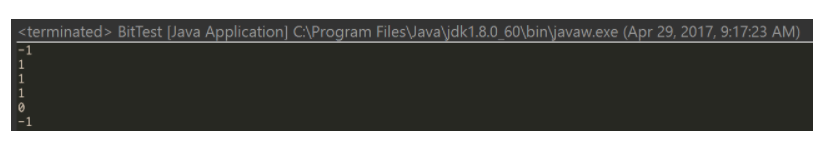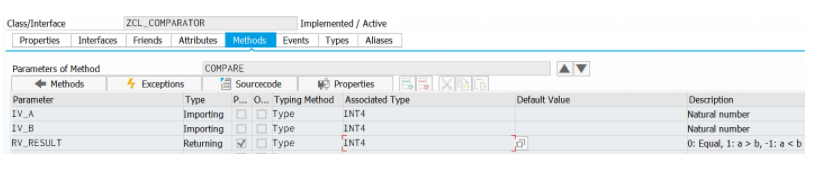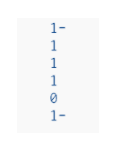ABAP面试问题 - 不使用加减乘除等操作比较两个整数大小

+关注继续查看

Our team architect has asked us this question which is said to be an interview question from Microsoft long time ago:

Please implement one function which accepts two integers as input and generate the following result accordingly:

If a > b, return 1,

if a = b, return 0,

if a < b, return -1For simplification reason here we can just consider unsigned int ( that is, all importing parameter of integers are greater than or equal to 0 ).

Inside the implementation, you are NOT allowed to use +, -, *, /, > and < for comparison.

There must be multiple ways to achieve it, here below is just one among them. Even we are not allowed to use four arithmetic operations and > or <, we can still leverage the bit operation supported on Integer.

The basic idea is, say we have 6 and 5 for comparison.

Binary format of 6: 0110

Binary format of 5: 0101If we can generate the biggest-sub-bits-series which differentiate the two integers, in the example above it is 0010( since the third bit of both integer are equal ), then we can simply know which is bigger by making bit AND operation:

0110 & 0010  = 10 which <> 0.

0101 & 0010 = 0So we can know 0110 > 0101.

Another example – compare 4 and 3Binary format of 4: 0100

Binary format of 3: 0011The biggest-sub-bits-series: 01000100 & 0100 = 0100 which <> 0

0011 & 0100 = 0So 0100 > 0011.

Solution in JavaScriptfunction compare(a,b){

var diff = a ^ b;

if( diff == 0)

return 0;

diff = diff | ( diff >> 1 );

diff |= diff >> 2;

diff |= diff >> 4;

diff |= diff >> 8;

diff |= diff >> 16;

diff ^= diff >> 1;

return  ( a & diff )? 1:-1;

}

console.log(compare(1,2));

console.log(compare(3,2));

console.log(compare(300,2));

console.log(compare(3000,2));

console.log(compare(3000,3000));

console.log(compare(3000,3001));Output:

Solution in Javapublic static int compare(int a, int b){

int diff = a ^ b;

if( diff == 0)

return 0;

diff = diff | ( diff >> 1 );

diff |= diff >> 2;

diff |= diff >> 4;

diff |= diff >> 8;

diff |= diff >> 16;

diff ^= diff >> 1;

return  ( (a & diff) == 0 )  ? -1 : 1;

}

System.out.println(compare(1,2));

System.out.println(compare(3,2));

System.out.println(compare(300,2));

System.out.println(compare(3000,2));

System.out.println(compare(3000,3000));

System.out.println(compare(3000,3001));Output:Solution in ABAP

Since it is not possible to directly perform bit operation on integer in ABAP, in my blog Bitwise operation ( OR, AND, XOR ) on ABAP Integer I simulate these three operations with the help of ABAP internal table. Still it is not enough, the bit shift operation like >> and << are also required to finish this exercise, so I make further enhancement, adding two new methods SHIFT_RIGHT and SHIFT_LEFT in ZCL_INTEGER, which could be found from my github.

Now all prerequisite to finish it using ABAP are fulfilled.

Here it is:Source code:METHOD compare.

DEFINE shift_right.

lv_diff = a->get_raw_value( ).

a->shift_right( &1 ).

lo_diff = zcl_integer=>value_of( lv_diff ).

a = lo_diff->or( a ).

END-OF-DEFINITION.

DATA(a) = zcl_integer=>value_of( iv_a ).

DATA(b) = zcl_integer=>value_of( iv_b ).

DATA: lv_diff TYPE int4,

lo_diff TYPE REF TO zcl_integer.

a = a->xor( b ).

IF a->get_raw_value( ) IS INITIAL.

rv_result = 0.

RETURN.

ENDIF.

shift_right 1.

shift_right 2.

shift_right 4.

shift_right 8.

shift_right 16.

lv_diff = a->get_raw_value( ).

a->shift_right( 1 ).

lo_diff = zcl_integer=>value_of( lv_diff ).

a = lo_diff->xor( a ).

DATA(lo_origin_a) = zcl_integer=>value_of( iv_a ).

rv_result = zcl_integer=>value_of( lo_origin_a->and( a )->get_raw_value( ) )->get_raw_value( ).

rv_result = COND #( WHEN rv_result IS INITIAL THEN -1 ELSE 1 ).

ENDMETHOD.Test code:WRITE:/ zcl_comparator=>compare( iv_a = 1 iv_B = 2 ).

WRITE:/ zcl_comparator=>compare( iv_a = 3 iv_B = 2 ).

WRITE:/ zcl_comparator=>compare( iv_a = 300 iv_B = 2 ).

WRITE:/ zcl_comparator=>compare( iv_a = 3000 iv_B = 2 ).

WRITE:/ zcl_comparator=>compare( iv_a = 3000 iv_B = 3000 ).

WRITE:/ zcl_comparator=>compare( iv_a = 3000 iv_B = 3001 ).Test output:Further reading

I have written a series of blogs which compare the language feature among ABAP, JavaScript and Java. You can find a list of them below:

Lazy Loading, Singleton and Bridge design pattern in JavaScript and in ABAP

Functional programming – Simulate Curry in ABAP

Functional Programming – Try Reduce in JavaScript and in ABAP

Simulate Mockito in ABAP

A simulation of Java Spring dependency injection annotation @Inject in ABAP

Singleton bypass – ABAP and Java

Weak reference in ABAP and Java

Fibonacci Sequence in ES5, ES6 and ABAP

Java byte code and ABAP Load

How to write a correct program rejected by compiler: Exception handling in Java and in ABAP

An small example to learn Garbage collection in Java and in ABAP

String Template in ABAP, ES6, Angular and React

Try to access static private attribute via ABAP RTTI and Java Reflection

Local class in ABAP, Java and JavaScript

Integer in ABAP, Java and JavaScript

Covariance in Java and simulation in ABAP

Various Proxy Design Pattern implementation variants in Java and ABAP

Tag(Marker) Interface in ABAP and Java

Bitwise operation ( OR, AND, XOR ) on ABAP Integer

An interview question: Compare two integers without +,-,*,/ or > and <[spring]spring中使用jackson返回字段(属性名)大小写格式化问题
2643 08643 0Backup Exec 16使用虚拟机方式增量备份SQL时备份大小异常的解决方法
789 0978 0Ubuntu中使用SSHSecure Shell测试Windows与Linux系统间操作及传输问题解决大全

1105 010472 019 012294 06074 0
2315

0

+ 订阅

《2021云上架构与运维峰会演讲合集》

《零基础CSS入门教程》

《零基础HTML入门教程》